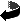# Dominant energy condition and spinors on Lorentzian manifolds by Bernd Ammann, Jonathan Glöckle

Dominant energy condition and spinors on Lorentzian manifolds (.pdf)

### Abstract

Let (N,h) be a time- and space-oriented Lorentzian spin manifold, and let M be a compact spacelike hypersurface of N with induced Riemannian metric g and second fundamental form K. If (N,h) satisfies the dominant energy condition in a strict sense, then the Dirac-Witten operator of M⊂ N is an invertible, self-adjoint Fredholm operator. This allows us to use index theoretical methods in order to detect non-trivial homotopy groups in the space of initial data pairs on \$M\$ satisfying the dominant energy condition in a strict sense. The central tool will be a Lorentzian analogue of Hitchin's α-invariant. In case that the dominant energy condition only holds in a weak sense, the Dirac-Witten operator may be non-invertible, and we will study the kernel of this operator in this case.
We will show that the kernel may only be non-trivial if π1(M) is virtually solvable of derived length at most 2. This allows to extend the index theoretical methods to spaces of initial data pairs, satisfying the dominant energy condition in the weak sense.

We will show further that a spinor φ is in the kernel of the Dirac--Witten operator on (M,g,K) if and only if (M,g,K,φ)\$ admits an extension to a Lorentzian manifold (N,h) with parallel spinor ψ such that M is a Cauchy hypersurface of (N,h), such that g and K are the induced metric and second fundamental form of M, respectively, and φ is the restriction of ψ to M.Back to my Homepage

Bernd Ammann, Impressum und Datenschutzerklärung